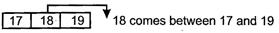Courses

# Test: Numbers Up to 20- 2

## 10 Questions MCQ Test Mathematics for Class 1 | Test: Numbers Up to 20- 2

Description
This mock test of Test: Numbers Up to 20- 2 for Class 1 helps you for every Class 1 entrance exam. This contains 10 Multiple Choice Questions for Class 1 Test: Numbers Up to 20- 2 (mcq) to study with solutions a complete question bank. The solved questions answers in this Test: Numbers Up to 20- 2 quiz give you a good mix of easy questions and tough questions. Class 1 students definitely take this Test: Numbers Up to 20- 2 exercise for a better result in the exam. You can find other Test: Numbers Up to 20- 2 extra questions, long questions & short questions for Class 1 on EduRev as well by searching above.
QUESTION: 1

### Study the pattern and choose the correct option? 50, 52, 54, 56 .......

Solution:

Skip counting of 2 will give this pattern 50, 52, 54, 56, 58, 60.

QUESTION: 2

### Rahul has 4 cricket balls and Lucky has 1 extra ball than Rahul. How many balls Lucky has in total?

Solution:
• Rahul has 4 balls.
• Lucky has 4 + 1 extra ball. He has 5 balls in total.
QUESTION: 3

### Which number comes after 5?

Solution:

Number 6 comes after number 5.

QUESTION: 4

Which of the following is an incorrect match?

Solution:

14 has 1 tens and 4 ones.

QUESTION: 5

I am a number between 20 and 50. In my tens place, I have the same number as the number of wheels in an auto-rickshaw. In my ones place, I have the same number as the number of wheels in a bicycle. What number am I?

Solution:

An auto-rickshaw has 3 wheels. So, number on tens place is 3 and a bicycle has 2 wheels, so numbers on ones place is 2. Therefore the number is 32.

QUESTION: 6

Which of the following number has 4 at tens place and 6 at ones place?

Solution:

4 tens and 6 ones make number 46.

QUESTION: 7

Which of the following statements is not true?

Solution:

26 > 62 is not true. 62 > 26. In 62, 6 is on tens place In 26, 2 is on tens place 6 > 2 => 62 > 26.

QUESTION: 8

One more than smallest two digit number is ______

Solution:

Smallest two digit-number is 10 and 1 more than 10 is 11.

QUESTION: 9

Which number comes between 17 and 19?

Solution:QUESTION: 10

Which of the following is true about number 10?

Solution:

10 is the smallest two digit number.